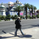TradingView39360 visningar
Another new indicator for TV community :)

STC detects up and down trends long before the MACD . It does this by using the same exponential moving averages (EMAs), but adds a cycle component to factor instrument cycle trends. STC gives more accuracy and reliability than the MACD .

More info: http://www.investopedia.com/articles/for...

Feel free to "Make mine" this chart and use the indicator in your charts. Appreciate any feedback on how effective this is for your instrument (I have tested this only with BTC ).

For people trading BTC:
-------------------------------

Try 3/10 or 9/30 for MACD (fastLength/slowLength). They seem to catch the cycles better than the defaults. :)
Open-source script

In true TradingView spirit, the author of this script has published it open-source, so traders can understand and verify it. Cheers to the author! You may use it for free, but reuse of this code in a publication is governed by House Rules. You can favorite it to use it on a chart.

Want to use this script on a chart?
```//
// @author LazyBear
// If you use modify / use this code, appreciate if you could drop me a note.
//
study(title="Schaff Trend Cycle [LazyBear]", shorttitle="STC_LB", overlay=true)
length=input(10)
fastLength=input(23)
slowLength=input(50)
macd(source, fastLength, slowLength) =>
fastMA = ema(source, fastLength)
slowMA = ema(source, slowLength)
macd = fastMA - slowMA
macd

stc(length, fastLength, slowLength) =>
factor=input(0.5)
m = macd(close,fastLength,slowLength)
v1 = lowest(m, length)
v2 = highest(m, length) - v1
f1 = (v2 > 0 ? ((m - v1) / v2) * 100 : nz(f1))
pf = (na(pf) ? f1 : pf + (factor * (f1 - pf)))
v3 = lowest(pf, length)
v4 = highest(pf, length) - v3
f2 = (v4 > 0 ? ((pf - v3) / v4) * 100 : nz(f2))
pff = (na(pff) ? f2 : pff + (factor * (f2 - pff)))
pff

plot(stc(length,fastLength,slowLength),color=red, title="Schaff_TC")
//
// Uncomment the lines below if you want the center region
//
// ul=plot(25)
// ll=plot(75)
// fill(ul,ll,color=red)

```
List of my free indicators: http://bit.ly/1LQaPK8
List of my indicators at Appstore: http://blog.tradingview.com/?p=970

## KommentarerYou can also use this indicator in its own pane (by unmerging down), as shown above.

If you do that, I suggest enabling the center region. Open the source code and uncomment these lines. Press Save and voila!

//
// ul=plot(25)
// ll=plot(75)
// fill(ul,ll,color=red)
//
SvaraCoinEx
I just tried this code, it worked.

Did you make any changes?
SvaraLazyBear
I thought that might have been a possibility. So what I did is I took a fresh copy from above and inserted it a few minutes ago. The error appears when you uncomment the last few lines and prevents the indicator from functioning.

In other words, it is a carbon copy of the original.
SvaraCoinEx
uncomment last few lines?

Can you post the exact src code that is giving u this issue?
SvaraLazyBear
//
// @author LazyBear
// If you use modify / use this code, appreciate if you could drop me a note.
//
study(title="Schaff Trend Cycle ", shorttitle="STC_LB", overlay=true)
length=input(10)
fastLength=input(23)
slowLength=input(50)
macd(source, fastLength, slowLength) =>
fastMA = ema(source, fastLength)
slowMA = ema(source, slowLength)
macd = fastMA - slowMA
macd

stc(length, fastLength, slowLength) =>
factor=input(0.5)
m = macd(close,fastLength,slowLength)
v1 = lowest(m, length)
v2 = highest(m, length) - v1
f1 = (v2 > 0 ? ((m - v1) / v2) * 100 : nz(f1))
pf = (na(pf) ? f1 : pf + (factor * (f1 - pf)))
v3 = lowest(pf, length)
v4 = highest(pf, length) - v3
f2 = (v4 > 0 ? ((pf - v3) / v4) * 100 : nz(f2))
pff = (na(pff) ? f2 : pff + (factor * (f2 - pff)))
pff

plot(stc(length,fastLength,slowLength),color=red, title="Schaff_TC")
//
// Uncomment the lines below if you want the center region
//
ul = plot(open)
ll = plot(75)
fill(uline, ll, color=red)

SvaraCoinEx
I did this but it gives me error. Could you please post the script with the detached panel please? Many thanks.
Thanks LazyBear for this!!!
Svara